# Problems of the volume

#### Number of problems found: 1076

• Sea waterSeawater contains about 4.3% salt. How many dm3 of distilled water we must pour into 5 dm3 of sea water to get water with 1.8% salt?
• Pillar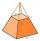Calculate volume of pillar shape of a regular tetrahedral truncated pyramid, if his square have sides a = 19, b = 27 and height is h = 48.
• Cone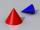If the segment of the line y = -3x +4, that lies in quadrant I, is rotated about the y-axis, a cone is formed. What is the volume of the cone?
• LatheFrom the cube of edge, 37 cm was a lathed maximum cylinder. What percentage of the cube is left as waste after lathed?
• Cube zoomHow many percents do we increase the volume and surface of the cube if we magnify its edge by 38 %?
• WaterMix 67 l of water with a temperature of 67 °C, 17 l warm of 32 °C, and 79 l water of 87 °C. What is the temperature of the mixed water immediately after mixing?
• CylindersThe area of the side of two cylinders is the same rectangle of 33 mm × 18 mm. Which cylinder has a larger volume and by how much?
• AlcoholHow many 55% alcohol we need to pour into 14 liters 75% alcohol to get p3% alcohol? How many 65% alcohol we get?
• Sea waterMixing 62 kg of sea water with 84 kg rainwater is created water containing 3.1% salt. How many percent sea water contains salt?
• Vinegar 2How many percentages of vinegar solution will we get if we mix 3.5 liters of 5.8% and 5 liters of 7.6% vinegar?
• Bath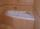In the bath is 30 liters of hot water. Then added 36 liters of cold water at temperature of 19 °C decreased temperature of water to 41 °C. What was the initial temperature of the hot water?
• Solution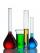In 469 dl red solution is 84 dl red color and in 102 dl blue solution is 52 dl blue color. How many dl of red and blue dl color solution must be mixed to get a mixture of 247 dl contain 116 dl of color?
• Spherical capFrom the sphere of radius 11 was truncated spherical cap. Its height is 6. What part of the volume is a spherical cap from the whole sphere?
• Rectangular cuboidThe rectangular cuboid has a surface area 5334 cm2, and its dimensions are in the ratio 2:4:5. Find the volume of this rectangular cuboid.
• 2x cone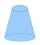Circular cone height 84 cm was cut plane parallel with base. Volume of these two small cones is the same. Calculate the height of the smaller cone.
• ConeCircular cone of height 15 cm and volume 5699 cm3 is at one-third of the height (measured from the bottom) cut by a plane parallel to the base. Calculate the radius and circumference of the circular cut.
• Cube in a sphereThe cube is inscribed in a sphere with a volume 7253 cm3. Determine the length of the edges of a cube.
• Vinegar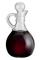We must dilute 16 liters of 8.8% aqueous vinegar to 4.1% one. How much water is necessary to add?
• Peroxide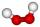How much distilled water (in liters) must pharmacists pour into 300 ml of 23.6% solution of hydrogen peroxide to get 2.7% solution to gargle?
• CuboidCuboid with edge a=6 cm and space diagonal u=31 cm has volume V=900 cm3. Calculate the length of the other edges.

Do you have an exciting math question or word problem that you can't solve? Ask a question or post a math problem, and we can try to solve it.

We will send a solution to your e-mail address. Solved examples are also published here. Please enter the e-mail correctly and check whether you don't have a full mailbox.